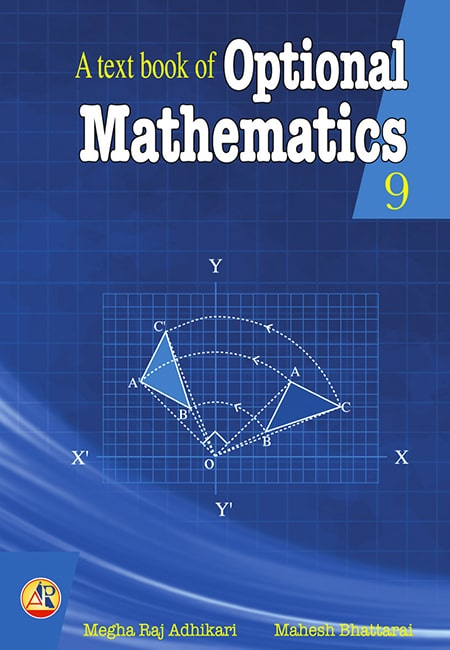## Optional Mathematics 9

Authors : Meghraj Adhikari, Mahesh Bhattarai

Features

• Strictly based on CDC curriculum
• Sufficient elaboration of each chapter
• Diagrammatically explained (as much as possible)
• Emphasis has been given to practical application
• 'Notes' are mentioned
• Formulae are summarized
• Suffiient examples are provided in each chapter
• Well-designed exercises
• Good coverage of the problems in each exercise